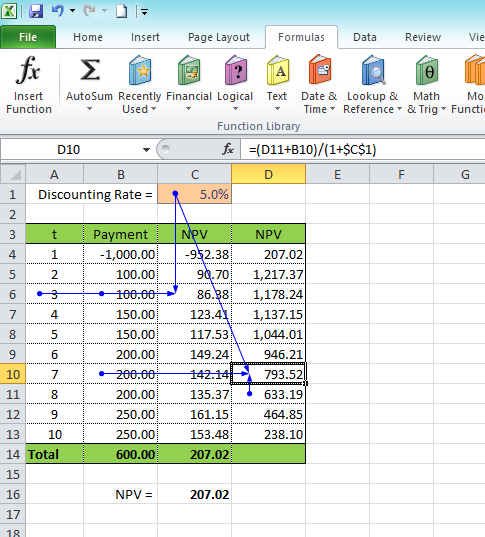# Calculating Net Present Value (NPV) using Excel

In finance, it is simply not enough to compare the total amount of money to estimate and evaluate cash flow. It is also highly significant to take into account a time interval of the cash flow.

As money might lose its value over time, the same period of time should be taken to compare certain amount of money. In this case, Net Present Value (NVP) is used as an initial time interval of the cash flow.

If Rt is a number of payments made at time t and at discount rate of i, thus the sum of money will be calculated based on the initial time interval (t=0):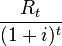The NPV formula for calculating the total cash flow will be:There is a function in Excel used for the NPV calculation:

`=NPV(discount_rate,payments_range)`

To better understand the whole process of NPV calculations, let's calculate the present value without using the Excel function. For example, we have 10 payments made at the discount rate of 5%:

•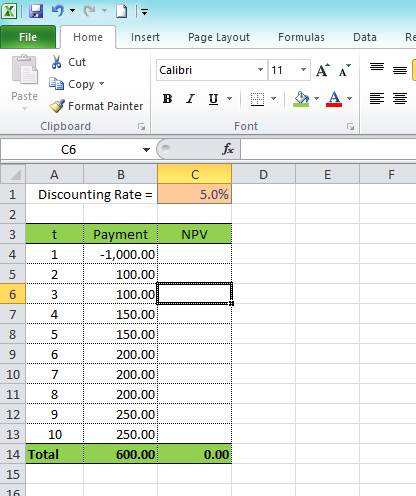In the NPV column we will calculate each payment for each period of time by using the following formula (cell C4):

`=B4/(1+\$C\$1)^A4`

If you copy the formula and apply it to the cells C5:C13, you will find its total sum and get its total value where NPV = 207.02. This value will be the same as the one you would get by calculations made according to the following formula

`=NPV(C1,B4:B13)`
•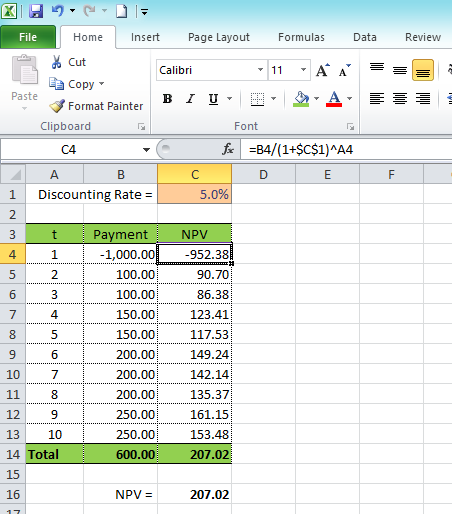During the NPV calculation, you might also avoid the calculation of power function for discount rates by using a recursive formula:

`NPV(n-1) = NPV(n) / (1+i)`

To better present the calculation method, let's add another column where the calculation will be made from bottom to top (from the cells D13 to D4). Use the formulas shown below:

```Cell D13: = B13/(1+\$C\$1)
Cell D12: = (D13+B12)/(1+\$C\$1)```

Copy the formula from the cell D12 in order to apply it for other cells D4:D11. In this case, D4 will contain the NPV.

If we have a look at the cells used in the first and second methods of calculations, we can see that the recursive method uses not only the current period of time, but also takes into account one period forward.

•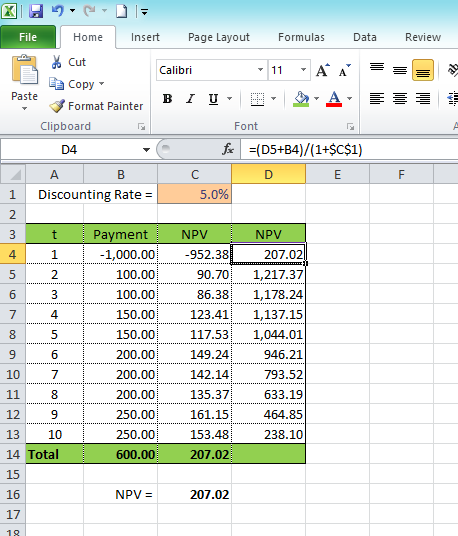•